# RD Sharma Solutions for Class 8 Maths Chapter 21 Mensuration - II (Volumes and Surface Areas of a Cuboid and a Cube) Exercise 21.2

So far we have used cubic centimetre as a standard unit of measurement of volume. We know cubic centimetre is a very small unit for measuring volumes of water tanks, oil tanks etc. In Exercise 21.2 of Chapter 21, we shall now discuss other units of measurements of volume. Students can make use of RD Sharma Class 8 Solutions to acquire more knowledge to solve the problems. Also, access RD Sharma solutions for Class 8 Maths Chapter 21 Exercise 21.2 PDF that is available below.

## Download the Pdf of RD Sharma Solutions for Class 8 Maths Exercise 21.2 Chapter 21 Mensuration – II (Volumes and Surface Areas of a Cuboid and a Cube)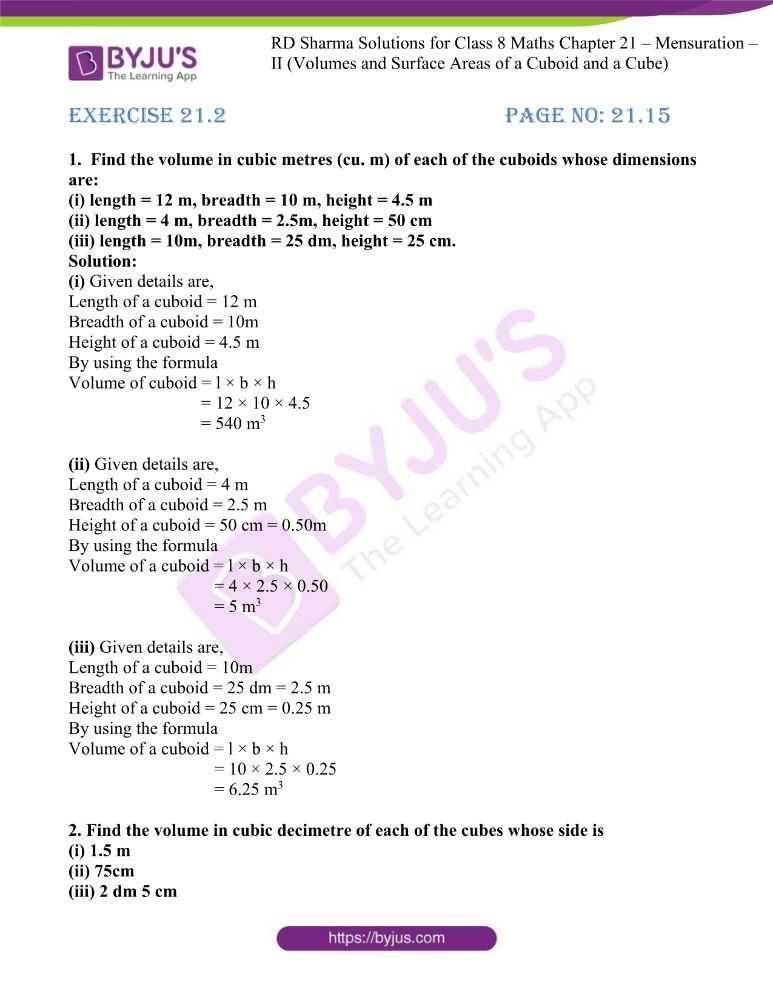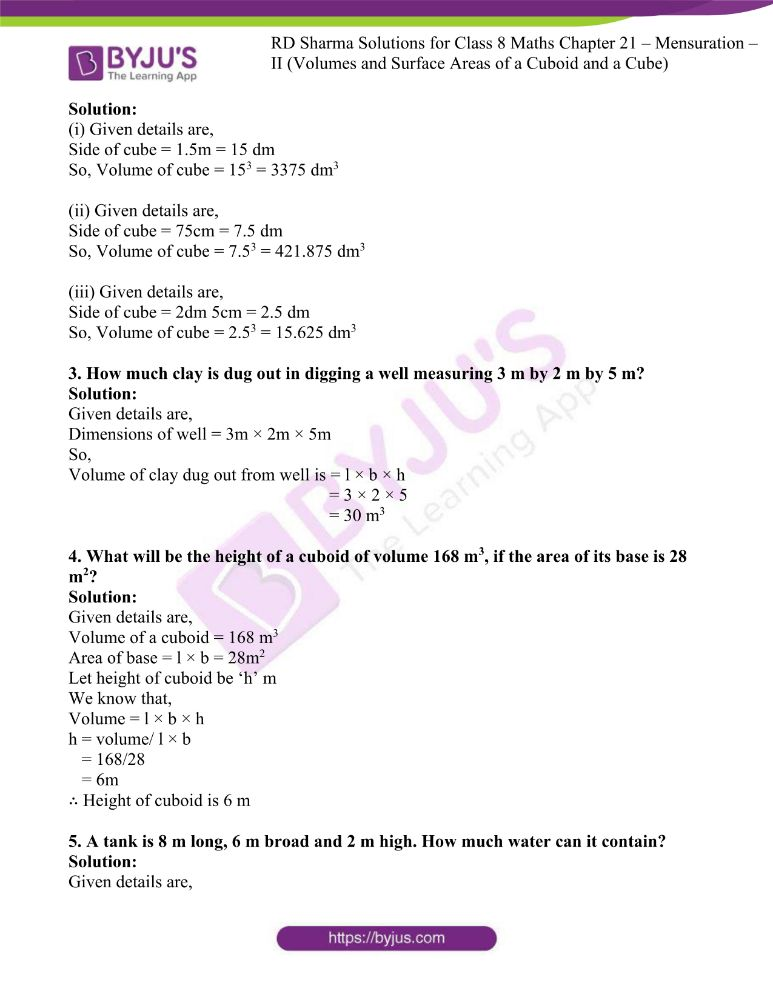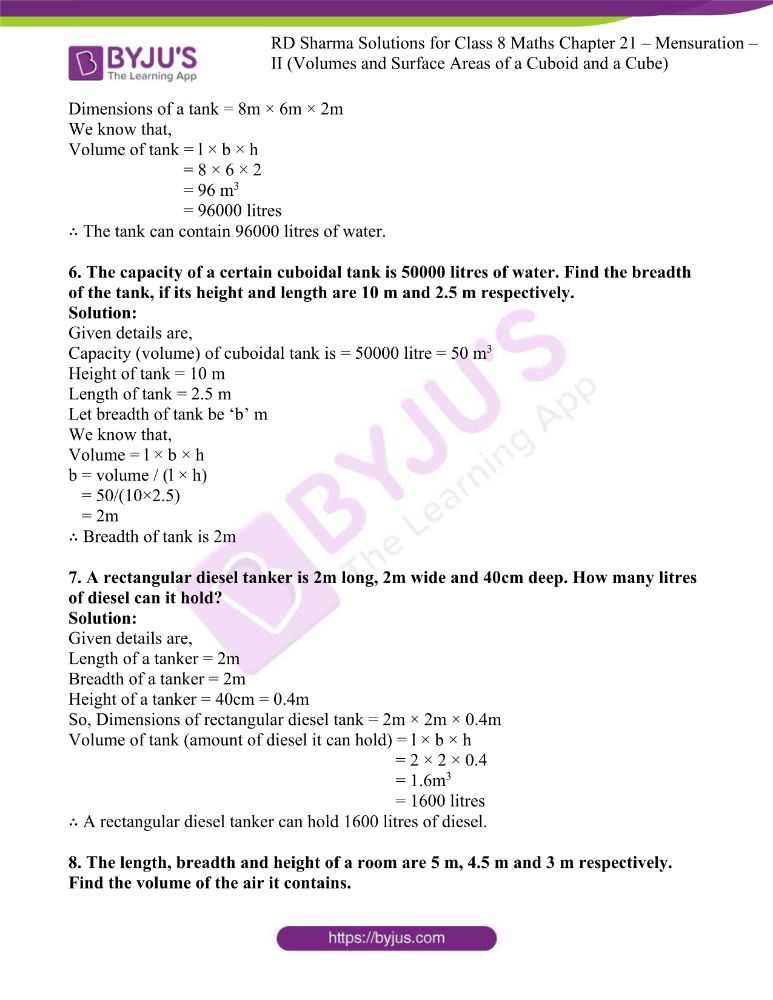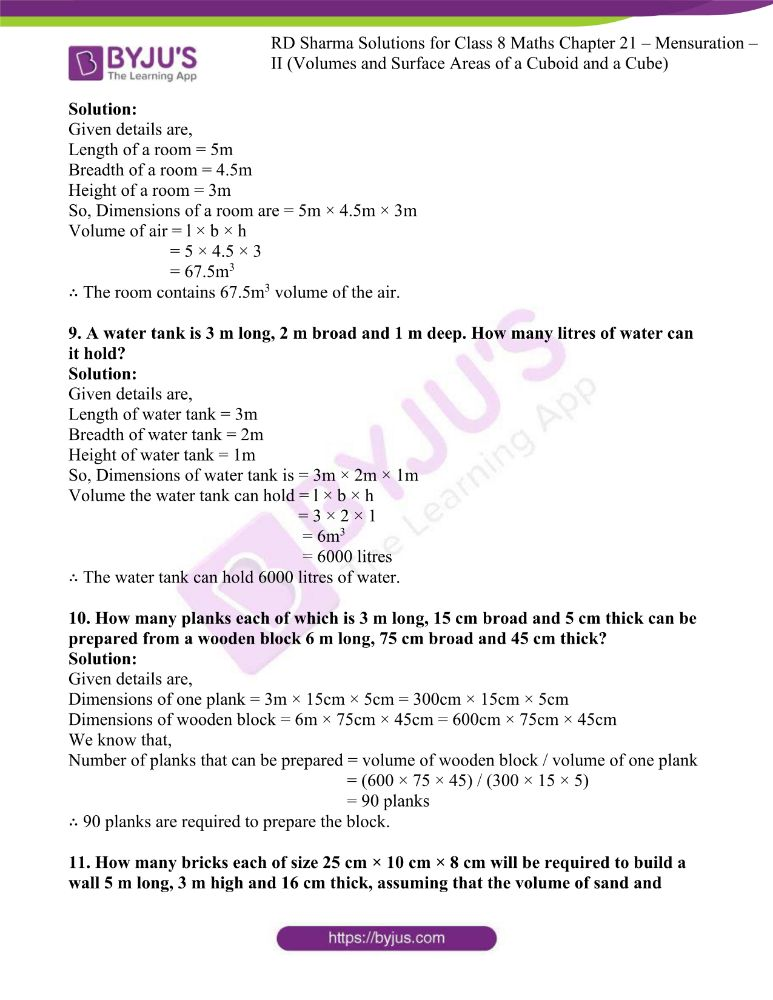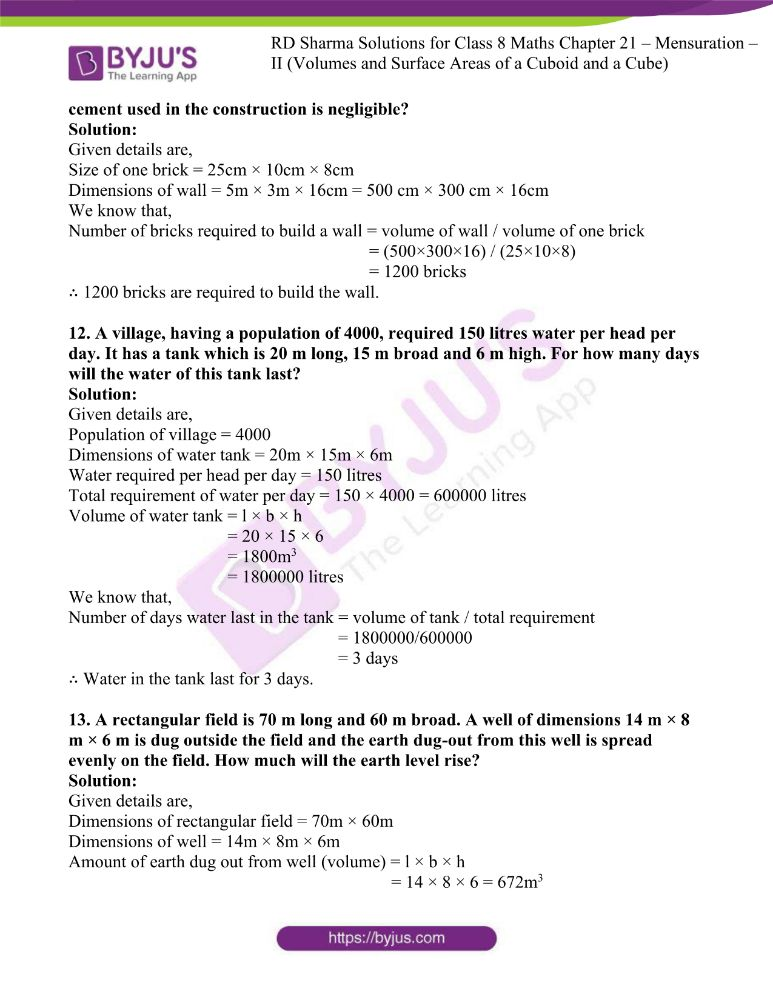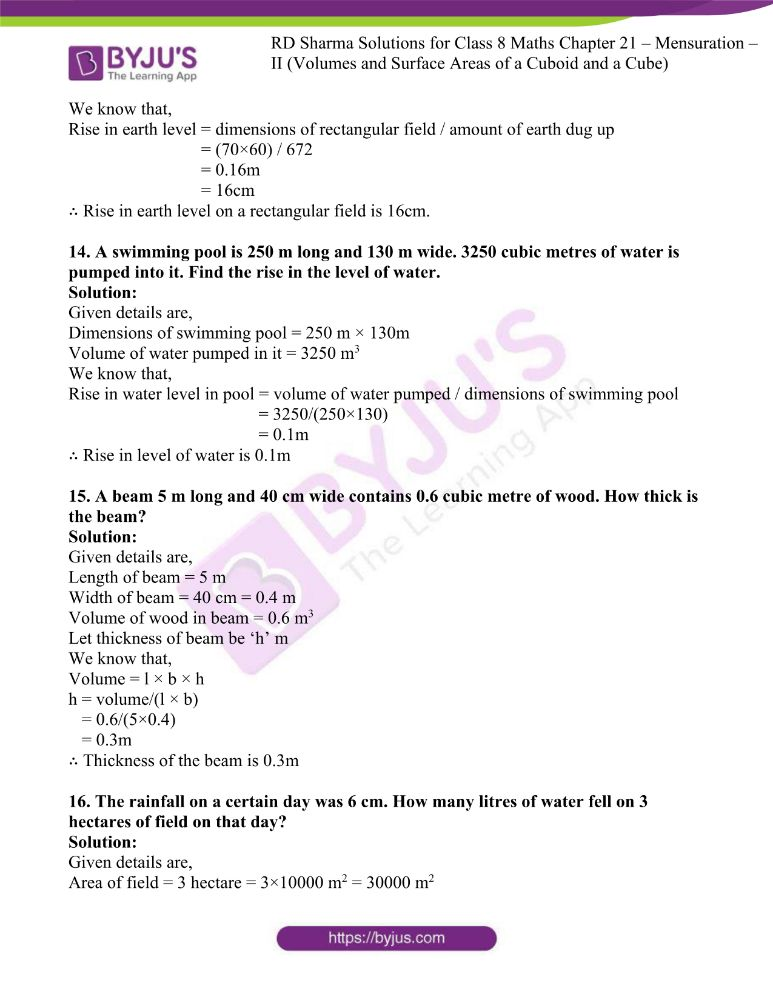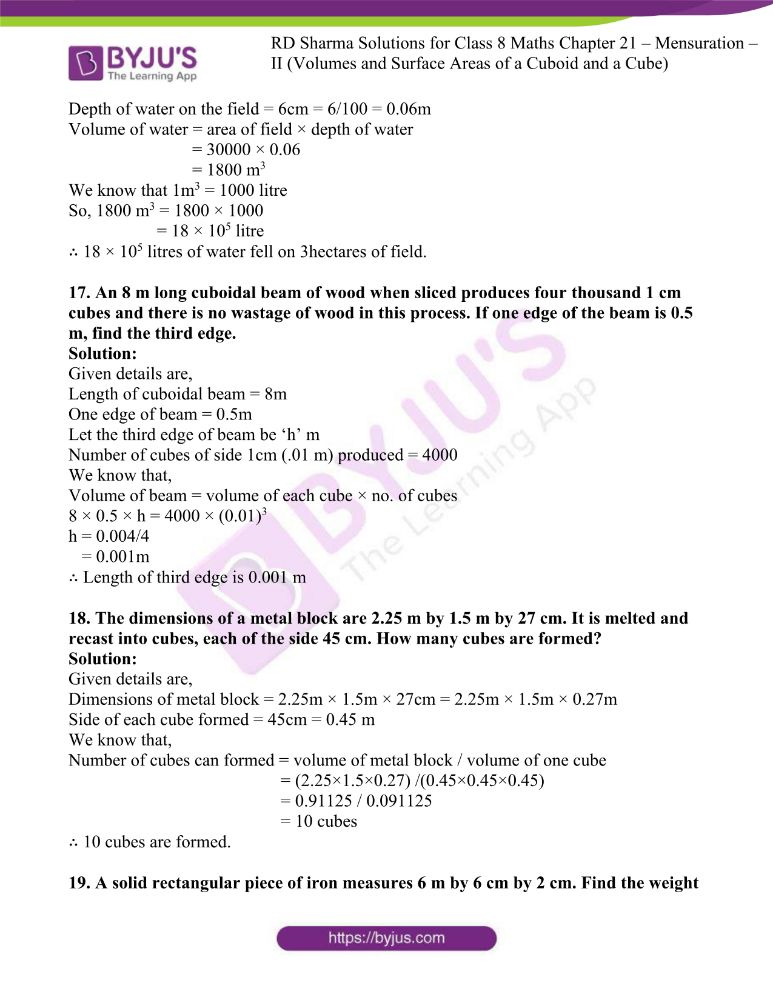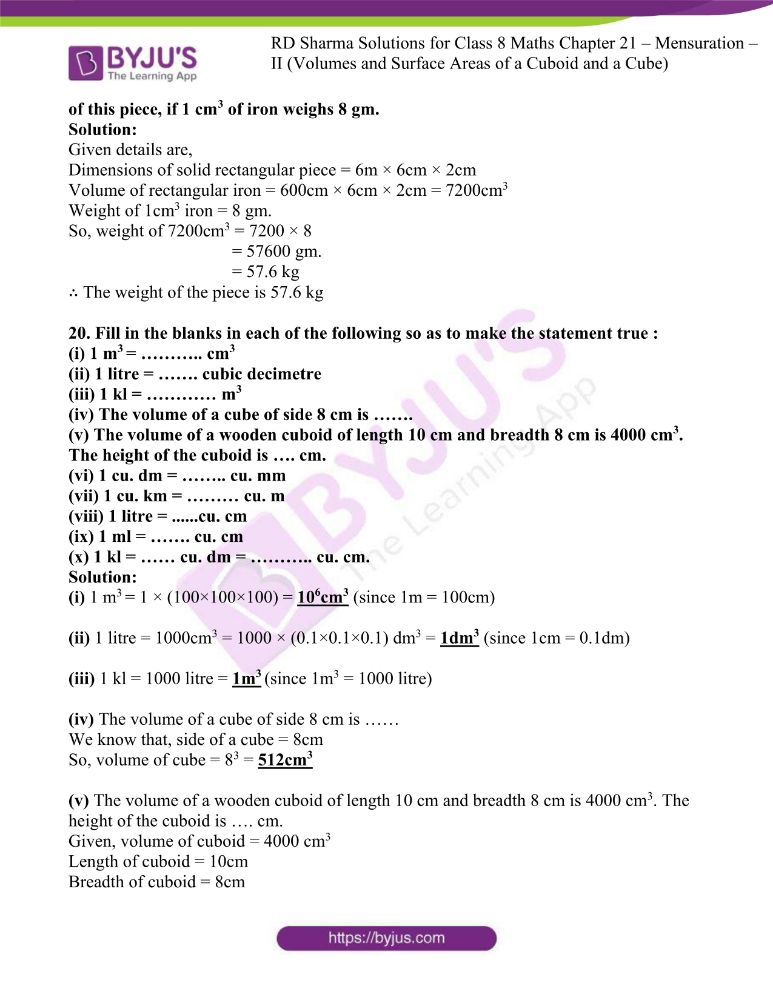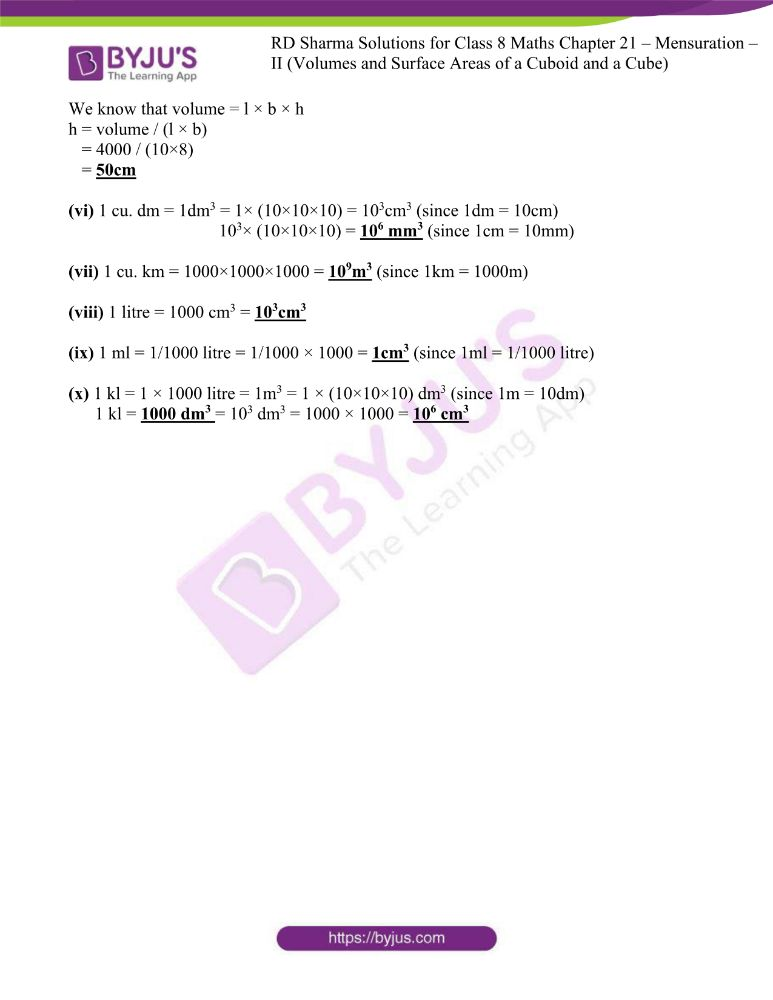### Access answers to RD Sharma Maths Solutions For Class 8 Exercise 21.2 Chapter 21 Mensuration – II (Volumes and Surface Areas of a Cuboid and a Cube)

1.  Find the volume in cubic metres (cu. m) of each of the cuboids whose dimensions are:
(i) length = 12 m, breadth = 10 m, height = 4.5 m
(ii) length = 4 m, breadth = 2.5m, height = 50 cm
(iii) length = 10m, breadth = 25 dm, height = 25 cm.

Solution:

(i) Given details are,

Length of a cuboid = 12 m

Breadth of a cuboid = 10m

Height of a cuboid = 4.5 m

By using the formula

Volume of cuboid = l × b × h

= 12 × 10 × 4.5

= 540 m3

(ii) Given details are,

Length of a cuboid = 4 m

Breadth of a cuboid = 2.5 m

Height of a cuboid = 50 cm = 0.50m

By using the formula

Volume of a cuboid = l × b × h

= 4 × 2.5 × 0.50

= 5 m3

(iii) Given details are,

Length of a cuboid = 10m

Breadth of a cuboid = 25 dm = 2.5 m

Height of a cuboid = 25 cm = 0.25 m

By using the formula

Volume of a cuboid = l × b × h

= 10 × 2.5 × 0.25

= 6.25 m3

2. Find the volume in cubic decimetre of each of the cubes whose side is
(i) 1.5 m

(ii) 75cm
(iii) 2 dm 5 cm

Solution:

(i) Given details are,

Side of cube = 1.5m = 15 dm

So, Volume of cube = 153 = 3375 dm3

(ii) Given details are,

Side of cube = 75cm = 7.5 dm

So, Volume of cube = 7.53 = 421.875 dm3

(iii) Given details are,

Side of cube = 2dm 5cm = 2.5 dm

So, Volume of cube = 2.53 = 15.625 dm3

3. How much clay is dug out in digging a well measuring 3 m by 2 m by 5 m?

Solution:

Given details are,

Dimensions of well = 3m × 2m × 5m

So,

Volume of clay dug out from well is = l × b × h

= 3 × 2 × 5

= 30 m3

4. What will be the height of a cuboid of volume 168 m3, if the area of its base is 28 m2?

Solution:

Given details are,

Volume of a cuboid = 168 m3

Area of base = l × b = 28m2

Let height of cuboid be ‘h’ m

We know that,

Volume = l × b × h

h = volume/ l × b

= 168/28

= 6m

∴ Height of cuboid is 6 m

5. A tank is 8 m long, 6 m broad and 2 m high. How much water can it contain?

Solution:

Given details are,

Dimensions of a tank = 8m × 6m × 2m

We know that,

Volume of tank = l × b × h

= 8 × 6 × 2

= 96 m3

= 96000 litres

∴ The tank can contain 96000 litres of water.

6. The capacity of a certain cuboidal tank is 50000 litres of water. Find the breadth of the tank, if its height and length are 10 m and 2.5 m respectively.

Solution:

Given details are,

Capacity (volume) of cuboidal tank is = 50000 litre = 50 m3

Height of tank = 10 m

Length of tank = 2.5 m

Let breadth of tank be ‘b’ m

We know that,

Volume = l × b × h

b = volume / (l × h)

= 50/(10×2.5)

= 2m

∴ Breadth of tank is 2m

7. A rectangular diesel tanker is 2m long, 2m wide and 40cm deep. How many litres of diesel can it hold?

Solution:

Given details are,

Length of a tanker = 2m

Breadth of a tanker = 2m

Height of a tanker = 40cm = 0.4m

So, Dimensions of rectangular diesel tank = 2m × 2m × 0.4m

Volume of tank (amount of diesel it can hold) = l × b × h

= 2 × 2 × 0.4

= 1.6m3

= 1600 litres

∴ A rectangular diesel tanker can hold 1600 litres of diesel.

8. The length, breadth and height of a room are 5 m, 4.5 m and 3 m respectively. Find the volume of the air it contains.

Solution:

Given details are,

Length of a room = 5m

Breadth of a room = 4.5m

Height of a room = 3m

So, Dimensions of a room are = 5m × 4.5m × 3m

Volume of air = l × b × h

= 5 × 4.5 × 3

= 67.5m3

∴ The room contains 67.5m3 volume of the air.

9. A water tank is 3 m long, 2 m broad and 1 m deep. How many litres of water can it hold?

Solution:

Given details are,

Length of water tank = 3m

Breadth of water tank = 2m

Height of water tank = 1m

So, Dimensions of water tank is = 3m × 2m × 1m

Volume the water tank can hold = l × b × h

= 3 × 2 × 1

= 6m3

= 6000 litres

∴ The water tank can hold 6000 litres of water.

10. How many planks each of which is 3 m long, 15 cm broad and 5 cm thick can be prepared from a wooden block 6 m long, 75 cm broad and 45 cm thick?

Solution:

Given details are,

Dimensions of one plank = 3m × 15cm × 5cm = 300cm × 15cm × 5cm

Dimensions of wooden block = 6m × 75cm × 45cm = 600cm × 75cm × 45cm

We know that,

Number of planks that can be prepared = volume of wooden block / volume of one plank

= (600 × 75 × 45) / (300 × 15 × 5)

= 90 planks

∴ 90 planks are required to prepare the block.

11. How many bricks each of size 25 cm × 10 cm × 8 cm will be required to build a wall 5 m long, 3 m high and 16 cm thick, assuming that the volume of sand and cement used in the construction is negligible?

Solution:

Given details are,

Size of one brick = 25cm × 10cm × 8cm

Dimensions of wall = 5m × 3m × 16cm = 500 cm × 300 cm × 16cm

We know that,

Number of bricks required to build a wall = volume of wall / volume of one brick

= (500×300×16) / (25×10×8)

= 1200 bricks

∴ 1200 bricks are required to build the wall.

12. A village, having a population of 4000, required 150 litres water per head per day. It has a tank which is 20 m long, 15 m broad and 6 m high. For how many days will the water of this tank last?

Solution:

Given details are,

Population of village = 4000

Dimensions of water tank = 20m × 15m × 6m

Water required per head per day = 150 litres

Total requirement of water per day = 150 × 4000 = 600000 litres

Volume of water tank = l × b × h

= 20 × 15 × 6

= 1800m3

= 1800000 litres

We know that,

Number of days water last in the tank = volume of tank / total requirement

= 1800000/600000

= 3 days

∴ Water in the tank last for 3 days.

13. A rectangular field is 70 m long and 60 m broad. A well of dimensions 14 m × 8 m × 6 m is dug outside the field and the earth dug-out from this well is spread evenly on the field. How much will the earth level rise?

Solution:

Given details are,

Dimensions of rectangular field = 70m × 60m

Dimensions of well = 14m × 8m × 6m

Amount of earth dug out from well (volume) = l × b × h

= 14 × 8 × 6 = 672m3

We know that,

Rise in earth level = dimensions of rectangular field / amount of earth dug up

= (70×60) / 672

= 0.16m

= 16cm

∴ Rise in earth level on a rectangular field is 16cm.

14. A swimming pool is 250 m long and 130 m wide. 3250 cubic metres of water is pumped into it. Find the rise in the level of water.

Solution:

Given details are,

Dimensions of swimming pool = 250 m × 130m

Volume of water pumped in it = 3250 m3

We know that,

Rise in water level in pool = volume of water pumped / dimensions of swimming pool

= 3250/(250×130)

= 0.1m

∴ Rise in level of water is 0.1m

15. A beam 5 m long and 40 cm wide contains 0.6 cubic metre of wood. How thick is the beam?

Solution:

Given details are,

Length of beam = 5 m

Width of beam = 40 cm = 0.4 m

Volume of wood in beam = 0.6 m3

Let thickness of beam be ‘h’ m

We know that,

Volume = l × b × h

h = volume/(l × b)

= 0.6/(5×0.4)

= 0.3m

∴ Thickness of the beam is 0.3m

16. The rainfall on a certain day was 6 cm. How many litres of water fell on 3 hectares of field on that day?

Solution:

Given details are,

Area of field = 3 hectare = 3×10000 m2 = 30000 m2

Depth of water on the field = 6cm = 6/100 = 0.06m

Volume of water = area of field × depth of water

= 30000 × 0.06

= 1800 m3

We know that 1m3 = 1000 litre

So, 1800 m3 = 1800 × 1000

= 18 × 105 litre

∴ 18 × 105 litres of water fell on 3hectares of field.

17. An 8 m long cuboidal beam of wood when sliced produces four thousand 1 cm cubes and there is no wastage of wood in this process. If one edge of the beam is 0.5 m, find the third edge.

Solution:

Given details are,

Length of cuboidal beam = 8m

One edge of beam = 0.5m

Let the third edge of beam be ‘h’ m

Number of cubes of side 1cm (.01 m) produced = 4000

We know that,

Volume of beam = volume of each cube × no. of cubes

8 × 0.5 × h = 4000 × (0.01)3

h = 0.004/4

= 0.001m

∴ Length of third edge is 0.001 m

18. The dimensions of a metal block are 2.25 m by 1.5 m by 27 cm. It is melted and recast into cubes, each of the side 45 cm. How many cubes are formed?

Solution:

Given details are,

Dimensions of metal block = 2.25m × 1.5m × 27cm = 2.25m × 1.5m × 0.27m

Side of each cube formed = 45cm = 0.45 m

We know that,

Number of cubes can formed = volume of metal block / volume of one cube

= (2.25×1.5×0.27) /(0.45×0.45×0.45)

= 0.91125 / 0.091125

= 10 cubes

∴ 10 cubes are formed.

19. A solid rectangular piece of iron measures 6 m by 6 cm by 2 cm. Find the weight of this piece, if 1 cm3 of iron weighs 8 gm.

Solution:

Given details are,

Dimensions of solid rectangular piece = 6m × 6cm × 2cm

Volume of rectangular iron = 600cm × 6cm × 2cm = 7200cm3

Weight of 1cm3 iron = 8 gm.

So, weight of 7200cm3 = 7200 × 8

= 57600 gm.

= 57.6 kg

∴ The weight of the piece is 57.6 kg

20. Fill in the blanks in each of the following so as to make the statement true :
(i) 1 m3 = ……….. cm3
(ii) 1 litre = ……. cubic decimetre
(iii) 1 kl = ………… m3
(iv) The volume of a cube of side 8 cm is …….
(v) The volume of a wooden cuboid of length 10 cm and breadth 8 cm is 4000 cm3. The height of the cuboid is …. cm.
(vi) 1 cu. dm = …….. cu. mm
(vii) 1 cu. km = ……… cu. m
(viii) 1 litre = ……cu. cm
(ix) 1 ml = ……. cu. cm
(x) 1 kl = …… cu. dm = ……….. cu. cm.

Solution:

(i) 1 m3 = 1 × (100×100×100) = 106cm3 (since 1m = 100cm)

(ii) 1 litre = 1000cm3 = 1000 × (0.1×0.1×0.1) dm3 = 1dm3 (since 1cm = 0.1dm)

(iii) 1 kl = 1000 litre = 1m3 (since 1m3 = 1000 litre)

(iv) The volume of a cube of side 8 cm is ……

We know that, side of a cube = 8cm

So, volume of cube = 83 = 512cm3

(v) The volume of a wooden cuboid of length 10 cm and breadth 8 cm is 4000 cm3. The height of the cuboid is …. cm.

Given, volume of cuboid = 4000 cm3

Length of cuboid = 10cm

We know that volume = l × b × h

h = volume / (l × b)

= 4000 / (10×8)

= 50cm

(vi) 1 cu. dm = 1dm3 = 1× (10×10×10) = 103cm3 (since 1dm = 10cm)

103× (10×10×10) = 106 mm3 (since 1cm = 10mm)

(vii) 1 cu. km = 1000×1000×1000 = 109m3 (since 1km = 1000m)

(viii) 1 litre = 1000 cm3 = 103cm3

(ix) 1 ml = 1/1000 litre = 1/1000 × 1000 = 1cm3 (since 1ml = 1/1000 litre)

(x) 1 kl = 1 × 1000 litre = 1m3 = 1 × (10×10×10) dm3 (since 1m = 10dm)

1 kl = 1000 dm3 = 103 dm3 = 1000 × 1000 = 106 cm3